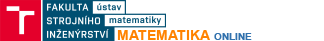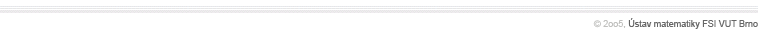Matrix Product - testingGenerates examples and check the result for the matrix product \$AB=C\$ including fractions.Matrix Product - calculationCalculation of the matrix product \$AB=C\$ for given \$A, B\$ including fractions.Inverse matrix - testingGenerates examples and check the result for the inverse matrix  \$A^(-1)=C\$ including fractions.Inverse matrix - calculationCalculation of the inverse matrix  \$A^(-1)=C\$ for a given matrix \$A\$ including fractions.Derivative of a function of one variable - testingGenerates examples and check the result for the derivative of a function of one variable \$f'(x)\$.Graphs of functions of one variableDraw graphs of several functions  \$y=f_1(x),  y=f_2(x), ...\$ in one picture.Parametrical curvesDraws a curve described by \$x=x(t), y=y(t)\$.Implicit curvesDraws a curve described by  \$f(x,y)=0\$.Couple of implicit curvesDraws two curves described by  \$f(x,y)=0, g(x,y)=0\$.The partial fraction decompositionThe partial fraction decomposition and antiderivative for the fraction of polynomials  \$f=(P_n)/(Q_m)\$.Taylor's   polynomialCalculates and draws the Taylor's polynomial for a entered function.